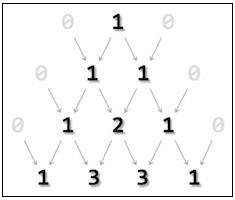# Java program to print Pascal's triangle

Pascal's triangle is one of the classic example taught to engineering students. It has many interpretations. One of the famous one is its use with binomial equations.

All values outside the triangle are considered zero (0). The first row is 0 1 0 whereas only 1 acquire a space in Pascal’s triangle, 0s are invisible. Second row is acquired by adding (0+1) and (1+0). The output is sandwiched between two zeroes. The process continues till the required level is achieved.## Algorithm

• Take a number of rows to be printed, n.
• Make outer iteration I for n times to print rows.
• Make inner iteration for J to (N - 1).
• Print single blank space " ".
• Close inner loop.
• Make inner iteration for J to I.
• Print nCr of I and J.
• Close inner loop.
• Print NEWLINE character after each inner iteration.

## Example

Live Demo

public class PascalsTriangle {
static int factorial(int n) {
int f;

for(f = 1; n > 1; n--){
f *= n;
}
return f;
}
static int ncr(int n,int r) {
return factorial(n) / ( factorial(n-r) * factorial(r) );
}
public static void main(String args[]){
System.out.println();
int n, i, j;
n = 5;

for(i = 0; i <= n; i++) {
for(j = 0; j <= n-i; j++){
System.out.print(" ");
}
for(j = 0; j <= i; j++){
System.out.print(" "+ncr(i, j));
}
System.out.println();
}
}
}

## Output

             1
1     1
1    2    1
1    3   3     1
1    4     6    4    1
1   5   10     10    5    1